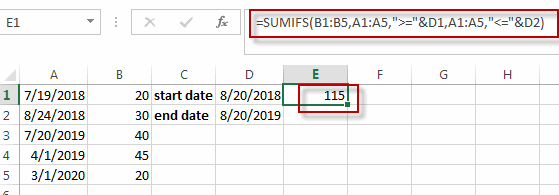# Sum Values between Two Dates

This post will guide you how to sum values in column where a date column meets two date criteria with a formula in Excel. How do I sum values between two dates in a range in Excel.

Assuming that you have two list of data in range A1:B5, and one column contain Dates and another column contain values. And you want to sum values in column B between two specified dates (2018/8/20-2019/8/20) in column A. How to achieve it. Let’s see the following detailed steps.

## Sum Values between Two Dates with Formula

If you want to quickly sum values between two dates in Excel, you can create a formula based on the SUMIF function. Like this:

`=SUMIFS(B1:B5,A1:A5,">="&D1,A1:A5,"<="&D2)`

Type this formula into a blank cell, and then press Enter key in your keyboard.You will see that the summed value has been generated.

### Related Functions

• Excel SUMIF Function
The Excel SUMIF function sum the numbers in the range of cells that meet a single criteria that you specify. The syntax of the SUMIF function is as below:=SUMIF (range, criteria, [sum_range])…